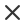FiltrTyp média
Typ licence
Orientace
Styl
Neobsahuje

# Fibonacci Reklamní vektory a ilustrace

Leonardo da vinci day the golden ratio template spiral fibonacci number circles in golden proportion icon mathematics with formula code drawing physics grid divine proportion line pattern geomeWhite line calculator icon isolated on black background accounting symbol business calculations mathematics education and finance vector illustrationPhysics education illustrations motion the laws of motion simple machines springs pulleys gears inclined planes next generation question template epsBeautiful math vector white graphs of eight petals rose grandi cardioid and silhouette of water lily flowers on blue backgroundMathematical vector seamless pattern with plots formulas geometrical figures and calculations you can use any color of backgroundManufacturing and industry illustration hand drawn sketch dimension of screw eye with self tapping used to attach cables to objectsSeamless linear pattern with school geometric elements pattern with mathematical objects figures pyramid cube ball cylinder vector background for geometrySacred geometry mathematics nature and spirituality in nature the formula of nature there is no beginning and no end of the universe and no beginning and no end of the life and the blissMath education vector pattern with handwritten formulas tasks plots calculations and geometrical figures vector illustrationLeonardo da vinci day the golden ratio template spiral fibonacci number circles in golden proportion icon mathematics with formula code drawing physics grid divine proportion line pattern geomePsychologist consulting or mental problem solving brainstorm concept two speech bubbles conversation icons with line scribbles coach mentoring psychotherapy complex to simple mind conceptAbstract futuristic thin line pattern with human silhouette and geometry shapes math science engineered drawn project plot conceptMathematical vector scientific technical seamless pattern with handwritten formulas calculations equations on a green backgroundMath linear mathematics education circle background with geometrical plots formulas and calculations design concept vector illustrationRealistic metal ruler vector markup for 10 inches and 25 centimeters rulers realistic metal ruler measuring tool metric precision double sided measuring instrumentMath education vector pattern with handwritten formulas tasks plots calculations and geometrical figures vector illustrationVector illustration of green apple with yellow measuring tape and circled arrows as concept of diet the tailor centimeter ribbon is wrapped around a fresh wet apple